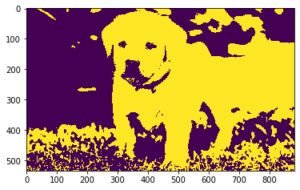# Mahotas – Closing Holes in Image

• Last Updated : 30 Jul, 2021

In this article we will see how we can close holes of the image in mahotas.  Closing holes means to remove the holes present in the image, closing is a process in which first dilation operation is performed and then erosion operation is performed. It eliminates the small holes from the obtained image, it is used for smoothening of contour and fusing of narrow breaks.

In this tutorial we will use “lena” image, below is the command to load it.

Attention geek! Strengthen your foundations with the Python Programming Foundation Course and learn the basics.

To begin with, your interview preparations Enhance your Data Structures concepts with the Python DS Course. And to begin with your Machine Learning Journey, join the Machine Learning - Basic Level Course

`mahotas.demos.load('lena')`

Below is the lena imageIn order to do this we will use mahotas.close_holes method

Syntax : mahotas.close_holes(img)

Argument : It takes image object as argument

Return : It returns image object

Note: Input image should be filtered or should be loaded as grey

In order to filter the image we will take the image object which is numpy.ndarray and filter it with the help of indexing, below is the command to do this

`image = image[:, :, 0]`

Below is the implementation

## Python3

 `# importing required libraries``import` `mahotas``import` `mahotas.demos``from` `pylab ``import` `gray, imshow, show``import` `numpy as np``  ` `# loading image``img ``=` `mahotas.demos.load(``'lena'``)``  ` `# filtering image``img ``=` `img.``max``(``2``)``  ` `# otsu method``T_otsu ``=` `mahotas.otsu(img)``   ` `# image values should be greater than otsu value``img ``=` `img > T_otsu``  ` `print``(``"Image threshold using Otsu Method"``)``  ` `# showing image``imshow(img)``show()`` `  `# closing holes``new_img ``=` `mahotas.close_holes(img)`  `print``(``"Closed Hole Image"``)` `# showing image``imshow(new_img)``show()`

Output :

`Image threshold using Otsu Method`Lena Otsu

`Closed Hole Image`Close Holes

Another example

## Python3

 `# importing required libraries``import` `mahotas``import` `numpy as np``from` `pylab ``import` `gray, imshow, show``import` `os`` ` `# loading image``img ``=` `mahotas.imread(``'dog_image.png'``)` `# filtering image``img ``=` `img[:, :, ``0``]``  ` `# otsu method``T_otsu ``=` `mahotas.otsu(img)  ``  ` `# image values should be greater than otsu value``img ``=` `img > T_otsu``  ` `print``(``"Image threshold using Otsu Method"``)``  ` `# showing image``imshow(img)``show()``  `  `# closing holes``new_img ``=` `mahotas.close_holes(img)`  `print``(``"Closed Hole Image"``)` `# showing image``imshow(new_img)``show()`

Output :

`Image threshold using Otsu Method``Closed Hole Image`My Personal Notes arrow_drop_up